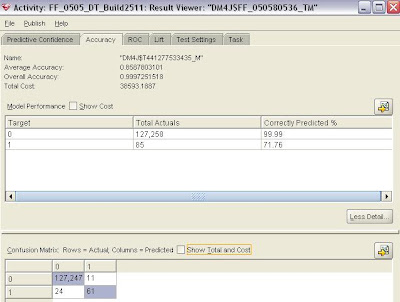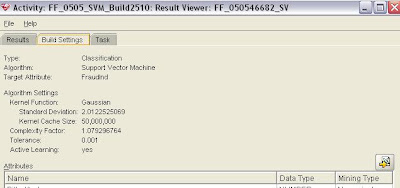# Fraud Prediction – Decision Trees & Support Vector MachinesNovember 25, 2008
632 Views

My first thought when I was asked to learn and use Oracle Data Mining (ODM) was, “Oh no! Yet another Data Mining Software!!!”

It’s been about 2 weeks now since I have been using ODM, particularly focusing on two classification techniques – Decision Trees & Support Vector Machines. As I don’t want to get into the details of the interface/usability of ODM (unless Oracle pays me!!), I will limit this post on a comparison of these two classificat

My first thought when I was asked to learn and use Oracle Data Mining (ODM) was, “Oh no! Yet another Data Mining Software!!!”

It’s been about 2 weeks now since I have been using ODM, particularly focusing on two classification techniques – Decision Trees & Support Vector Machines. As I don’t want to get into the details of the interface/usability of ODM (unless Oracle pays me!!), I will limit this post on a comparison of these two classification techniques at a very basic level, using ODM.

A very brief introduction of DT & SVM.

DT – A flow chart or diagram representing a classification system or a predictive model. The tree is structured as a sequence of simple questions. The answers to these questions trace a path down the tree. The end product is a collection of hierarchical rules that segment the data into groups, where a decision (classification or prediction) is made for each group.

-The hierarchy is called a tree, and each segment is called a node.
-The original segment contains the entire data set, referred to as the root node of the tree.
-A node with all of its successors forms a branch of the node that created it.
-The final nodes (terminal nodes) are called leaves. For each leaf, a decision is made and applied to all observations in the leaf.

SVM – A Support Vector Machine (SVM) performs classification by constructing an N-dimensional hyperplane that optimally separates the data into two categories.

In SVM jargon, a predictor variable is called an attribute, and a transformed attribute that is used to define the hyperplane is called a feature. A set of features that describes one case/record is called a vector. The goal of SVM modeling is to find the optimal hyperplane that separates clusters of vector in such a way that cases with one category of the target variable are on one side of the plane and cases with the other category are on the other size of the plane. The vectors near the hyperplane are the support vectors.

SVM is a kernel-based algorithm. A kernel is a function that transforms the input data to a high-dimensional space where the problem is solved. Kernel functions can be linear or nonlinear.

The linear kernel function reduces to a linear equation on the original attributes in the training data. The Gaussian kernel transforms each case in the training data to a point in an n-dimensional space, where n is the number of cases. The algorithm attempts to separate the points into subsets with homogeneous target values. The Gaussian kernel uses nonlinear separators, but within the kernel space it constructs a linear equation.

I worked on this dataset which has fraudulent fuel card transactions. Two techniques I previously tried are Logistic Regression (using SAS/STAT) & Decision Trees (using SPSS Answer Tree). Neither of them was found to be suitable for this dataset/problem.

The dataset has about 300,000 records/transactions and about 0.06% of these have been flagged as fraudulent. The target variable is the fraud indicator with 0s as non-frauds, and 1s as frauds.

The Data Preparation consisted of missing value treatments, normalization, etc. Predictor variables that are strongly associated with the fraud indicator – both from the business & statistics perspective – were selected.

The dataset was divided into a Build Data (60% of the records) and Test Data (40% of the records).

Algorithm Settings for DT,Accuracy/Confusion Matrix for DT,Algorithm Settings for SVM,Accuracy/Confusion Matrix for SVM,